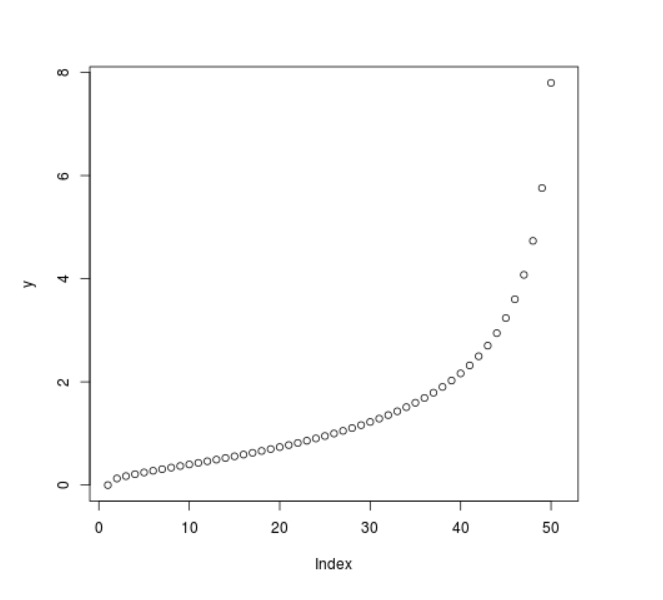# Compute value of Log Normal Quantile Function in R Programming – qlnorm() Function

`qlnorm()` function in R Language is used to compute the value of log normal quantile function. It also creates a plot of the quantile function of log normal distribution.

Syntax: qlnorm(vec)

Parameters:
vec: x-values for normal density

Example 1:

 `# R program to compute value of ` `# log normal quantile function ` ` `  `# Creating x-values for density ` `x <``-` `seq(``0``, ``1``, by ``=` `0.2``) ` ` `  `# Calling qlnorm() function ` `y <``-` `qlnorm(x) ` `y `

Output:

``` 0.0000000 0.4310112 0.7761984 1.2883304 2.3201254       Inf
```

Example 2:

 `# R program to compute value of ` `# log normal quantile function ` ` `  `# Creating x-values for density ` `x <``-` `seq(``0``, ``1``, by ``=` `0.02``) ` ` `  `# Calling qlnorm() function ` `y <``-` `qlnorm(x) ` ` `  `# Plot a graph ` `plot(y) `

Output:My Personal Notes arrow_drop_upCheck out this Author's contributed articles.

If you like GeeksforGeeks and would like to contribute, you can also write an article using contribute.geeksforgeeks.org or mail your article to contribute@geeksforgeeks.org. See your article appearing on the GeeksforGeeks main page and help other Geeks.

Please Improve this article if you find anything incorrect by clicking on the "Improve Article" button below.

Article Tags :

Be the First to upvote.

Please write to us at contribute@geeksforgeeks.org to report any issue with the above content.